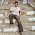Support us .Net Basics C# SQL ASP.NET Aarvi MVC Slides C# Programs Subscribe Download

### Part 56 - C# Tutorial - Generics in C#

Generics are introduced in C# 2.0. Generics allow us to design classes and methods decoupled from the data types. Generic classes are extensively used by collection classes available in System.Collections.Generic namespace. Click here to watch the video on generic collection classes.

In this example, AreEqual(int value1, int value2) only works with int data type. If, we pass any other data type, we get a compiler error. So, AreEqual() method in Calculator class is tightly coupled with the int data type, and prevents it from being used with any other data type.

Part 56 - C# Tutorial - Generics in C#

using System;
namespace Pragim
{
public class MainClass
{
private static void Main()
{
bool Equal = Calculator.AreEqual(1, 2);
if (Equal)
{
Console.WriteLine("Equal");
}
else
{
Console.WriteLine("Not Equal");
}
}
}
public class Calculator
{
public static bool AreEqual(int value1, int value2)
{
return value1 == value2;
}
}
}

It's a compile time error to invoke AreEqual() method with string parameters.
bool Equal = Calculator.AreEqual("A", "B");

One way of making AreEqual() method reusable, is to use object type parameters. Since, every type in .NET directly or indirectly inherit from System.Object type, AreEqual() method works with any data type, but the problem is performance degradation due to boxing and unboxing happening.

Also, AreEuqal() method is no longer type safe. It is now possible to pass integer for the first parameter, and a string for the second parameter. It doesn't really make sense to compare strings with integers.
using System;
namespace Pragim
{
public class MainClass
{
private static void Main()
{
bool Equal = Calculator.AreEqual("A", "B");
if (Equal)
{
Console.WriteLine("Equal");
}
else
{
Console.WriteLine("Not Equal");
}
}
}
public class Calculator
{
public static bool AreEqual(object value1, object value2)
{
return value1 == value2;
}
}
}

So, the probem with using System.Object type is that
1. AreEqual() method is not type safe
2. Performance degradation due to boxing and unboxing.

Both of these issues can be solved with generics and still make AreEqual() method work with different data types. The re written example using generics is shown below.
using System;
namespace Pragim
{
public class MainClass
{
private static void Main()
{
bool Equal = Calculator.AreEqual<int>(2, 1);
if (Equal)
{
Console.WriteLine("Equal");
}
else
{
Console.WriteLine("Not Equal");
}
}
}
public class Calculator
{
public static bool AreEqual<T>(T value1, T value2)
{
return value1.Equals(value2);
}
}
}

To make AreEqual() method generic, we specify a type parameter using angular brackets as shown below.
public static bool AreEqual<T>(T value1, T value2)

At the point, When the client code wants to invoke this method, they need to specify the type, they want the method to operate on. If the user wants the AreEqual() method to work with integers, they can invoke the method specifying int as the datatype using angular brackets as shown below.
bool Equal = Calculator.AreEqual<int>(2, 1);

To operate with string data type
bool Equal = Calculator.AreEqual<string>("A", "B");

In this example, we made the method generic. Along the same lines, it is also possible to make classes, interfaces and delegates generic.

1.Very Simple and easy explanation as usual. Kaha se itna talent laye ho. Please teach us also, how u learn each and every concept and explain them so easily. I wish I could understand and explain it in that way. Really Thanks a lot.

1.By Experience and hard working

2.Thanks a lot Mr. Venkat. You are c# guru.

3.public class Calculator
{
public static bool AreEqual(object value1, object value2)
{
return value1 == value2;
}
}

the above code is not working whn we pass integer as parameters,.. its returning always false.

1.When you compare two objects, the comparison is done on references(consider this as addresses).

As the reference/address of two different objects can never be the same,
you will always get "FALSE" even if the objects hold same or different values.

2.sir..in generic can we add any two int datatype variables
suppose
return value1+value2;

4.